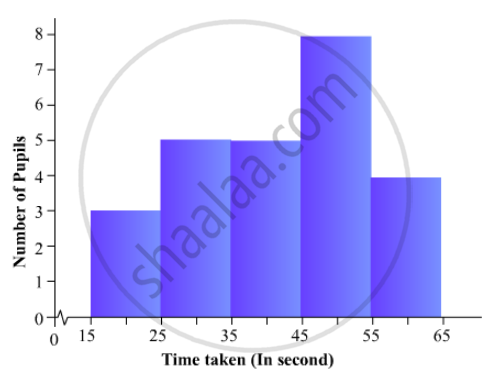# The Time Taken, in Seconds, to Solve a Problem by Each of 25 Pupils is as Follows: 16, 20, 26, 27, 28, 30, 33, 37, 38, 40, 42, 43, 46, 46, 46, 48, 49, 50, 53, 58, 59, 60, 64, 52, 20 - Mathematics

The time taken, in seconds, to solve a problem by each of 25 pupils is as follows:
16, 20, 26, 27, 28, 30, 33, 37, 38, 40, 42, 43, 46, 46, 46, 48, 49, 50, 53, 58, 59, 60, 64, 52, 20
(a) Construct a frequency distribution for these data, using a class interval of 10 seconds.
(b) Draw a histogram to represent the frequency distribution.

#### Solution

Given that the times (in seconds) taken to solve a problem by each of 25 pupils are 16, 20, 26, 27, 28, 30, 33, 37, 38, 40, 42, 43, 46, 46, 46, 48, 49, 50, 53, 58, 59, 60, 64, 52 and 20. The minimum and maximum time values are 16 and 64 respectively.

(a) At first construct the following frequency distribution for the given data. Since, the lowest value is 16; we start with the class-interval 15-25, as the class size must be 10.

 Class - Intervals Tally Frequency 15-25 lll 3 25-35 lllll 5 35-45 lllll 5 45-55 llllllll 8 55-65 llll 4

(b) To represent the given data by a histogram, we first draw horizontal and vertical axes. Let us consider that the horizontal and vertical axes represent the class-limits and the frequencies of the class-intervals respectively.

The above data is a continuous grouped frequency distribution with equal class-intervals, which is 10. Construct rectangles with class-intervals as bases and respective frequencies as heights.

The histogram of the data in part (a) is as follows:Concept: Graphical Representation of Data
Is there an error in this question or solution?

#### APPEARS IN

RD Sharma Mathematics for Class 9
Chapter 23 Graphical Representation of Statistical Data
Exercise 23.3 | Q 3 | Page 41

Share